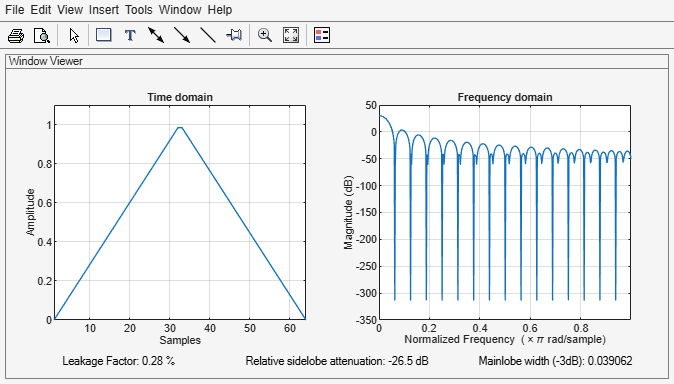# bartlett

Bartlett window

## Syntax

w = bartlett(L)

## Description

example

w = bartlett(L) returns an L-point symmetric Bartlett window.

## Examples

collapse all

Create a 64-point Bartlett window. Display the result using wvtool.

L = 64; bw = bartlett(L); wvtool(bw)## Input Arguments

collapse all

Window length, specified as a positive integer.

Data Types: single | double

## Output Arguments

collapse all

Bartlett window, returned as a column vector.

## Algorithms

The following equation generates the coefficients of a Bartlett window:

$w\left(n\right)=\left\{\begin{array}{ll}\frac{2n}{N},\hfill & 0\le n\le \frac{N}{2},\hfill \\ 2-\frac{2n}{N},\hfill & \frac{N}{2}\le n\le N.\hfill \end{array}$

The window length $L=N+1$.

The Bartlett window is very similar to a triangular window as returned by the triang function. However, the Bartlett window always has zeros at the first and last samples, while the triangular window is nonzero at those points. For odd values of L, the center L-2 points of bartlett(L) are equivalent to triang(L-2).

Note

If you specify a one-point window (L = 1), the value 1 is returned.

 Oppenheim, Alan V., Ronald W. Schafer, and John R. Buck. Discrete-Time Signal Processing. Upper Saddle River, NJ: Prentice Hall, 1999, pp.